Next: Subcritical Cloud vs Supercritical Up: Virial Analysis Previous: Virial Analysis   Contents

## Magnatohydrostatic Clouds

Consider here the effect of the magnetic field. In the magnetized medium, the Lorentz force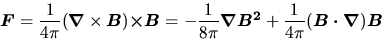(4.27)

works in the ionized medium. The first term of equation (4.27), which is called the magnetic pressure, has an effect to support the cloud against the self-gravity.

The virial analysis is also applicable to the magnetohydrostatic clouds. The terms related to the magnetic fields are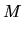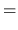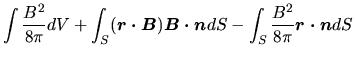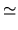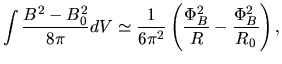(4.28)

where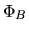represents a magnetic flux and it is assumed to be conserved if we change the radius,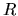, that is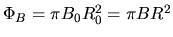. Equation (4.23) becomes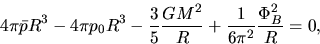(4.29)

where we ignored the term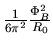. The last two terms are rewritten as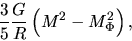(4.30)

where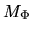is defined as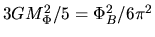.

This shows the effects of the magnetic fields:

1. B-fields effectively reduce the gravitational mass as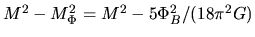. This plays a part to support a cloud.
2. However, even a cloud contracts (decreasing its radius from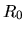to), the ratio of the gravitational to the magnetic terms keeps constant since these two terms are proportional to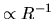. Thus, if the magnetic term does not work initially, the gravitational term continues to predominate over the magnetic term.
If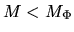, a sum of last two terms in equation (4.29) is positive. Since the second term of rhs of equation (4.25) is positive, there is onewhich satisfies equation (4.29) irrespective of the external pressure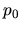. While, if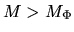, there is a maximum allowable external pressure. Therefore,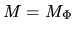gives a criterion whether the magnetic fields work to support the cloud or not. More realistic calculation [Mouschovias (1976a,1976b), Tomisaka et al (1988)] gives us a criterion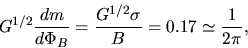(4.31)

where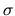and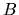means the column density and the magnetic flux density. A cloud with a mass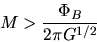(4.32)

is sometimes called magnetically supercritical, while that with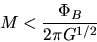(4.33)

is magnetically subcritical.

More precisely speaking, the criterion showed in equations (4.32) and (4.33) should be applied for a cloud which has a much larger mass than the Bonnor-Ebert mass. That is, even without magnetic fields, the cloud less-massive than the Bonnor-Ebert mass has a hydrostatic configuration shown in Figure 4.2 (left). The cloud with central density of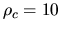has a stable density distribution. To fit the numerical results, Tomisaka et al (1988) obtained an expression for the critical mass when the cloud has a mass-to-flux ratio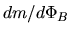, the isothermal sound speed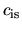, and the external pressureas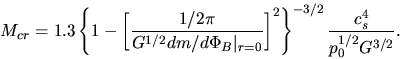(4.34)

This shows that the critical mass is a decreasing function of the mass-to-flux ratio or increasing function of the magnetic flux. And the critical mass becomes much larger than the Bonnor-Ebert mass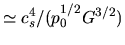only when the mass-to-flux ratio at the center of the cloud is reaching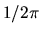at which the term in the curry bracket goes to zero. Hereafter, we call here the cloud/cloud core with mass larger than the critical mass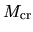a supercritical cloud/cloud core. The cloud/cloud core less-massive than the critical mass is subcritical.Next: Subcritical Cloud vs Supercritical Up: Virial Analysis Previous: Virial Analysis   Contents
Kohji Tomisaka 2007-07-08